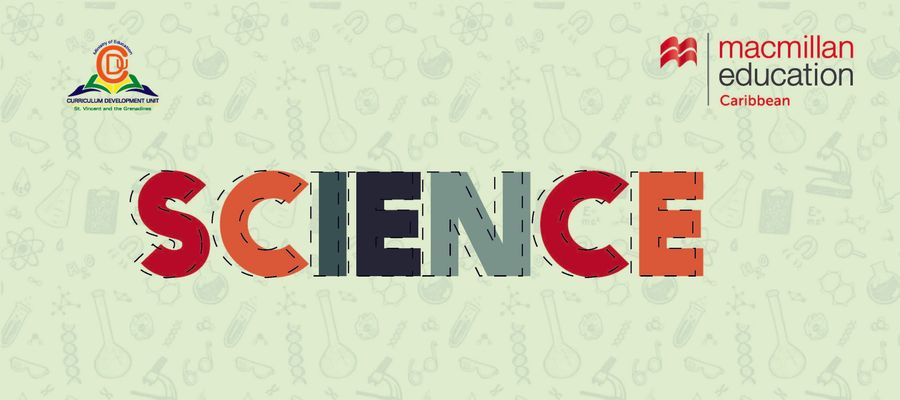# CPEA MOCK SCIENCE MCQ 7### Quiz Questions

• 1.
Which letter labelled the "INNER CORE"
• A.
B
• B.
D
• C.
C
• 2.
Earth is the _____planet from the sun.
• A.
second
• B.
third
• C.
fourth
• 3.
The hottest planet in the solar system is
• A.
Sun
• B.
Mercury
• C.
Venus
• 4.
What type of magnet is shown below?
• A.
flat magnet
• B.
bar magnet
• C.
horseshoe magnet
• 5.
The term pulls together in reference to magnets means
• A.
the magnetics attract each other
• B.
the magnets repel each other
• C.
the magnet attacks each other
• 6.
The end points of a magnet are called
• A.
charges
• B.
resistors
• C.
poles
• 7.
There are _______-temporary teeth.
• A.
14
• B.
20
• C.
32
• 8.
The part of the tooth above the gum is called
• A.
the dentine
• B.
the crown
• C.
root
• 9.
Which of the following is an  invertebrate
• A.
spider
• B.
lizard
• C.
bird
• 10.
Electrical energy can be transformed into
• A.
light energy
• B.
solar energy
• C.
nuclear energy

• 1.
Result 1